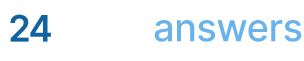# MATLAB for Computer Science

## Homework Help & Tutoring

We offer an array of different online MATLAB for Computer Science tutors, all of whom are advanced in their fields and highly qualified to instruct you.Send your subject help request Submit your homework problem, or a general tutoring request.
Get quotes from qualified tutors Receive a response from one of our tutors as soon as possible, sometimes within minutes!
MATLAB for Computer Science Tutors Available Now
28 tutors available
MATLAB for Computer Science Homework Library
69 total solutions
See what our students are saying
Can you help me with my homework in less than 24 hours?
Can you help me with my exam/quiz/test?
How much will it cost?
What kind of payments do you accept?

# MATLAB for Computer Science

Introduction to MATLAB

History

Many engineering and scientific challenges incur the need to organize data in matrices, perform various operations on them, and calculate the solution of a set of equations. The combination of more complex problems and evolving computer power led to the development of a special program for working on matrices called MATLAB (Matrix Laboratory). Work on MATLAB was initiated as far back as in the 1950s but the first real version called Fortran MATLAB was on the market in the early 1970s.1  The success of the early MATLAB releases enabled the founders to start a company in 1984 especially for handling MATLAB called the MathWorks2. Today, numerous university departments and corporations in most parts of the world are using it.

Basics

Everything in MATLAB is centered on the concept of a matrix. Strictly speaking, this is a group of numbers organized in a rectangular m x n collection, where the numbers may represent virtually anything. Basically, MATLAB treats scalars and vectors as special cases of matrices.

Operations

A number of ways to manipulate the data may become necessary, such as:

• Transposition
• Inverse if possible
• Multiplication
• Eigenvalue / Eigenvector calculation
• LU factorization
• Diagonalization

All of these are readily available in MATLAB. Strong built-in routines avoid loops and make for easier development.

Performance

While MATLAB offers strong built-in routines with fast computation, it lacks the optimization feature that platform specific HPC compilers give. This means that MATLAB code is generally slightly slower than optimized Fortran or C++ code, for example. Table 1 and 2 summarize the performance of MATLAB compared to a few contemporary computing environments for a number of arbitrary problems.

a. Double loop , 5000 x 5000 operations:

 Environment Computation time / s Optimized Fortran 0.26 NumPy 0.96 MATLAB 2.40 Java 12.6

Table 1. Performance for nested loop3

b) Matrix multiplication, 1500 x 1500

 Environment Computation time / s MATLAB 0.97 NumPy 0.96 Optimized Fortran 2.40 Java 12.6

Table 2. Performance for Matrix multiplication3

The tables illustrate how MATLABs’s strength lies in the built-in procedures rather than procedural programming. In Table 1, MATLAB is well behind Fortran and NumPy for a problem in the size of 20-30 million operations. However, Table 2 shows how MATLAB can outperform Fortran when the built-in matrix multiplication function is utilized.

Plotting

MATLAB also features a number of plotting options, in 2D and 3D. Lines can be in different shapes and colors, and data points emphasized in different ways. Plotting and visualizations are some of MATLAB’s great strengths.

References

1. Moler, C.: The Origins of MATLAB, http://se.mathworks.com/company/newsletters/articles/the-origins-of-matlab.html
2. The Mathworks, mathworks.com
3. Kouatchou, J.: Comparing Python, NumPy, Matlab, Fortran etc.

## College MATLAB for Computer Science Homework Help

Since we have tutors in all MATLAB for Computer Science related topics, we can provide a range of different services. Our online MATLAB for Computer Science tutors will:

• Provide specific insight for homework assignments.
• Review broad conceptual ideas and chapters.
• Simplify complex topics into digestible pieces of information.
• Answer any MATLAB for Computer Science related questions.
• Tailor instruction to fit your style of learning.

With these capabilities, our college MATLAB for Computer Science tutors will give you the tools you need to gain a comprehensive knowledge of MATLAB for Computer Science you can use in future courses.

## 24HourAnswers Online MATLAB for Computer Science Tutors

Our tutors are just as dedicated to your success in class as you are, so they are available around the clock to assist you with questions, homework, exam preparation and any MATLAB for Computer Science related assignments you need extra help completing.

In addition to gaining access to highly qualified tutors, you'll also strengthen your confidence level in the classroom when you work with us. This newfound confidence will allow you to apply your MATLAB for Computer Science knowledge in future courses and keep your education progressing smoothly.

Because our college MATLAB for Computer Science tutors are fully remote, seeking their help is easy. Rather than spend valuable time trying to find a local MATLAB for Computer Science tutor you can trust, just call on our tutors whenever you need them without any conflicting schedules getting in the way.

Start Working With Our College MATLAB for Computer Science Tutors
To fulfill our tutoring mission of online education, our college homework help and online tutoring centers are standing by 24/7, ready to assist college students who need homework help with all aspects of MATLAB for Computer Science.24houranswers.com Parker Paradigms, Inc 5 Penn PLaza, 23rd Floor New York, NY 10001
SUBJECTS COVERED